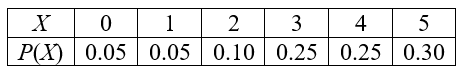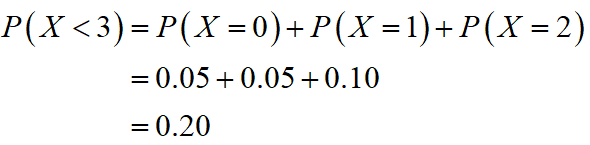# Javier volunteers in community events each month. He does not do more than five events in a month. He attends exactly five events 30% of the time, four events 25% of the time, three events 25% of the time, two events 10% of the time, one event 5% of the time, and no events 5% of the time.(a) Find the probability that Javier volunteers for less than three events each month.P(X < 3) = (b) Find the expected number of events Javier volunteers in a month. dont round

Question
55 views

Javier volunteers in community events each month. He does not do more than five events in a month. He attends exactly five events 30% of the time, four events 25% of the time, three events 25% of the time, two events 10% of the time, one event 5% of the time, and no events 5% of the time.

(a) Find the probability that Javier volunteers for less than three events each month.

P(X < 3) =

(b) Find the expected number of events Javier volunteers in a month. dont round

check_circle

Step 1

The probability distribution representing the number of volunteers X made by Javier in a month is,Step 2

(a)

The probability that Javier volunteers for less than three events each month is,Thus, the probability that Javier volunteers for less than three events each month is 0.20.

...

### Want to see the full answer?

See Solution

#### Want to see this answer and more?

Solutions are written by subject experts who are available 24/7. Questions are typically answered within 1 hour.*

See Solution
*Response times may vary by subject and question.
Tagged in